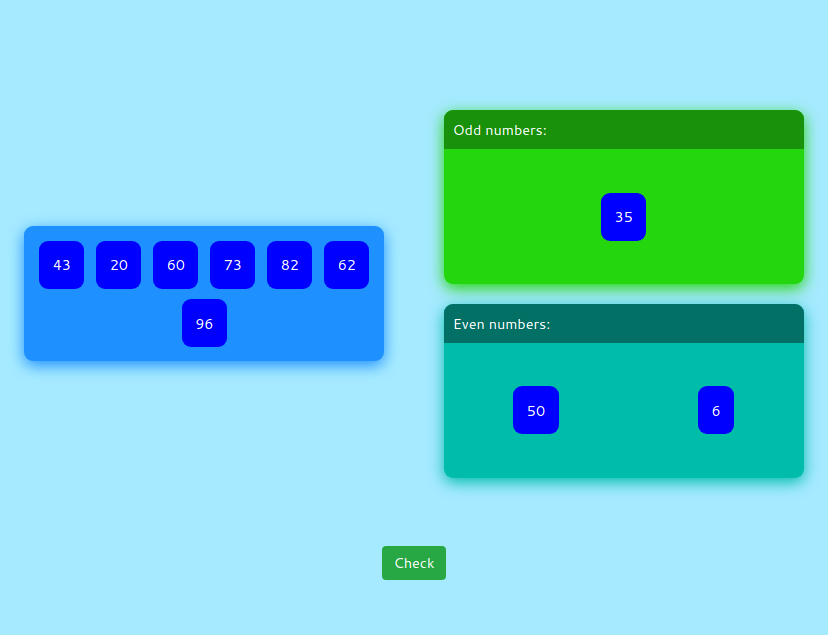MM Practice
×
Multiplication
Division
Place Value
Fractions & Decimals
Measurement
Statistics & Probability
Pre-Algebra
Money
Kindergarten
Geometry

# Even or Odd Numbers — Online Game (grades K-3)

Practice even and odd numbers by dragging each kind of number to its own "box" in this simple game.

You can choose the range of the numbers to sort. For example, for kindergarten children, choose a number range from 0 to 10 or 1 to 10 (single-digit numbers). First graders can practice with two-digit numbers (number range from 0 to 100), and so on.

Screenshot:Allow my comment to be posted on this site